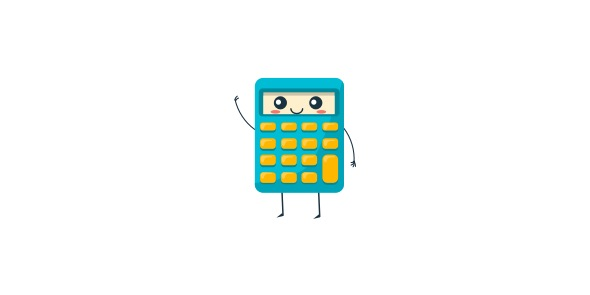5 Questions | Total Attempts: 2908SettingsDuring this year of the battle of the books seven books we have covered during the period between 2011 and 2012. How well do you remember each book we covered then? Take up the practice test below and refresh your mind by going back in time. All the best remembering!

Related Topics
• 1.
What are the proper names for these 2 figures?
• A.

Line AB and line GH

• B.

Line segment AB and line segment GH

• C.

• 2.
What is a 4 sided shape called?
• A.

Triangle

• B.

• C.

Pentagon

• 3.
What tranformation happened if a shape was flipped?
• A.

Rotation

• B.

Reflection

• C.

Translation

• 4.
On a coordinate plane, what is the point called where the two axes meet?
• A.

X-axis

• B.

Y-axis

• C.

Origin

• 5.
A triangle that has no equal sides and no equal angles is called what?
• A.

Equilateral

• B.

Iscoceles

• C.

Scalene